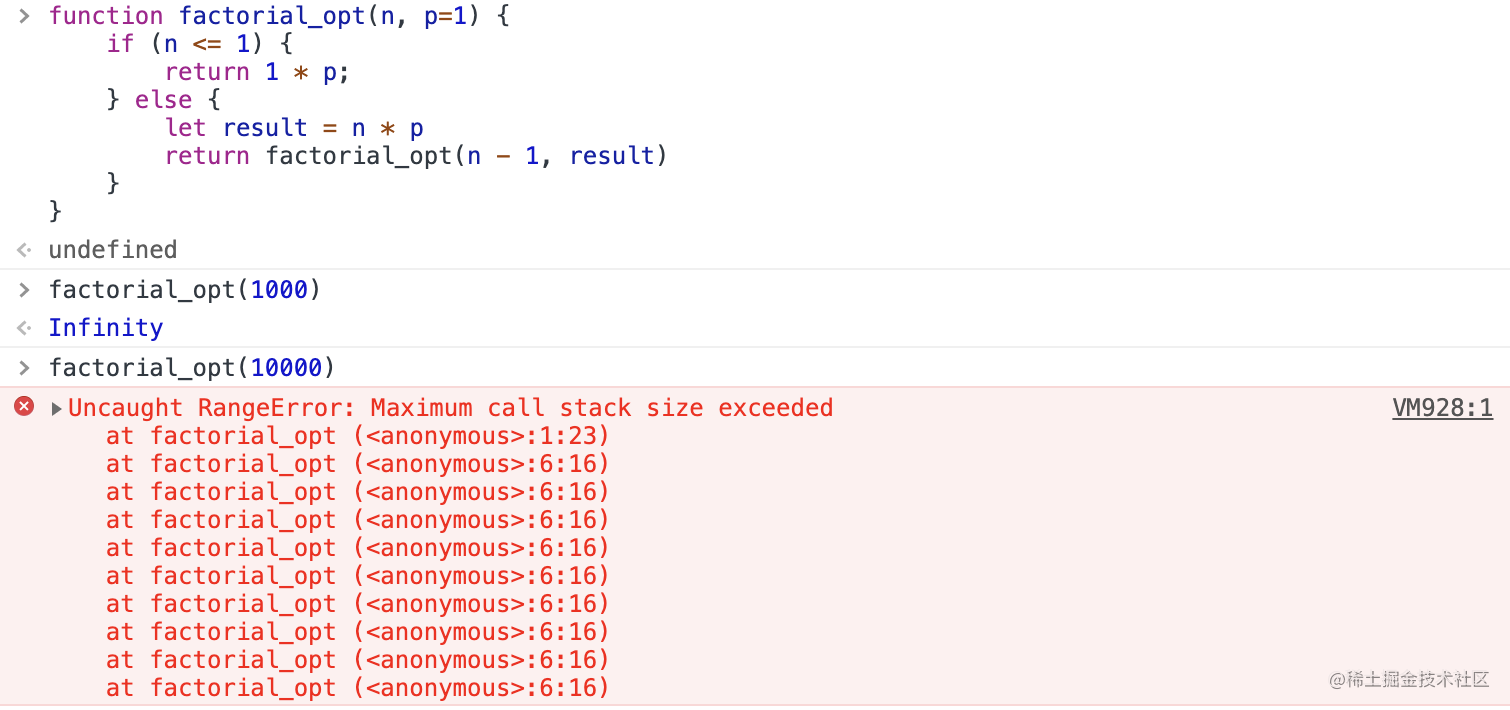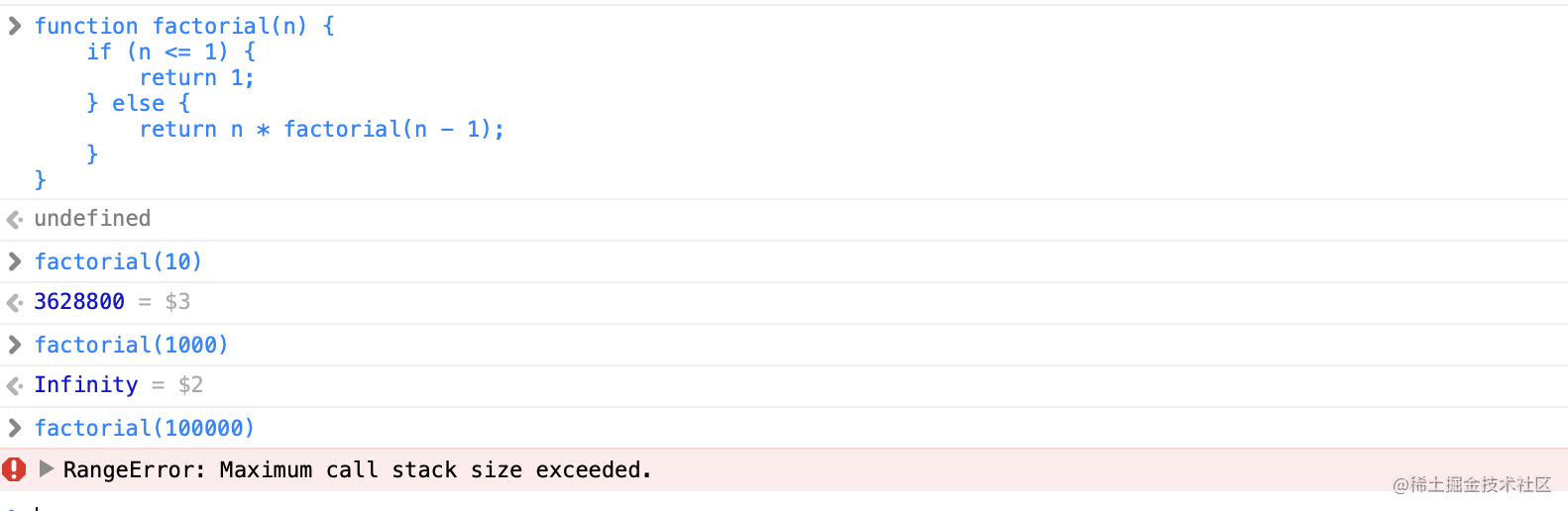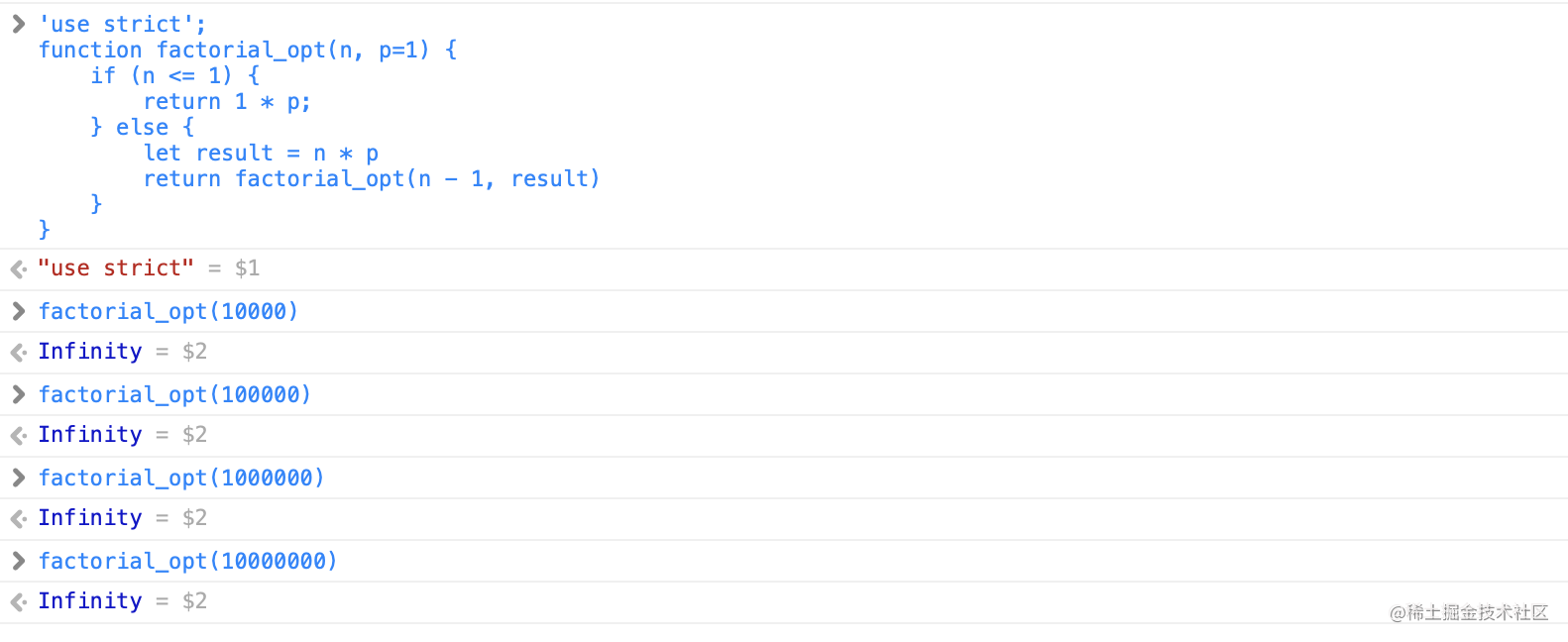# ES6尾调用优化

## 写在前面

ES6中函数比较有趣的变化有尾调用系统的引擎优化。尾调用是指函数作为另一个函数的最后一条语句被调用，就像这样

``````function doSomething() {
return doSomethingElse();
}
``````

ES5中在执行 doSomethingElse 函数的时候会新增一个栈帧，doSomething对应的栈帧会被保留在内存中，当存在循环调用时就容易出现程序问题，比如栈溢出等不可预期的错误。

## ES6中尾调用

### ES6中的尾调用优化的条件

• 严格模式下。
• 尾调用不访问当前栈帧的变量（也就是说函数不是一个闭包）
• 在函数内部，尾调用是最后一条语句。
• 尾调用的结果作为函数值返回。

``````"use strict";

// 可以被优化
function doSomething(){
／／优化后
return doSomethingElse();
}

// 无法优化，必须在返回值后添加其他操作
function doSomething(){
return 1+doSomethingElse();
}

// 无法优化，调用不在尾部
function doSomething(){
var result = doSomethingElse();
return result;
}

// 无法优化，func为闭包
function doSomething(){
var num=1, func = () => num;
return func();
}

``````

### 尾调用的案例

``````
function factorial(n) {
if (n <= 1) {
return 1;
} else {
return n * factorial(n - 1);
}
}
``````

``````'use strict';
function factorial_opt(n, p=1) {
if (n <= 1) {
return 1 * p;
} else {
let result = n * p
return factorial_opt(n - 1, result)
}
}
``````

### 验证结果 （一）``````\$ node -v
v10.24.1

\$ node foo.js
/Users/shuai/workspace/foo.js:2
function factorial_opt(n, p=1) {
^
RangeError: Maximum call stack size exceeded
at factorial_opt (/Users/shuai/workspace/foo.js:2:23)
...
``````

V8 曾经实现过尾调用优化，但后来又放弃了，主要是因为实现尾调用优化意味着要在代码执行的过程中修改调用栈，这样的话，执行流的信息就不完整了，而且还会导致两个问题：

debug 的时候调用栈信息不完整 error.stack 的信息会不完整 鉴于这些原因，V8 团队建议修改规范，把 PTC(Proper Tail Calls) 改为 STC(Syntatic Tail Calls)，也就是要用特定的语法来使用尾调用，比如 return continue func()。目前 V8 还是不支持的，曾经它提供了 --harmony-tailcalls 和 --harmony-explicit-tailcalls 来开启尾调用优化，不过后来又移除了。

### 验证结果 （二）

• 未优化的执行结果• 优化后的执行结果### 斐波那契数列

``````// 一般的实现方式
function fibonacci(n) {
if(n==0 || n == 1)
return n;
return fibonacci(n-1) + fibonacci(n-2);
}

// 尾调用优化后
'use strict'
function fibonacci_opt(n, current = 0, next = 1) {
if(n <= 1) {
return next
}
return fibonacci_opt(n - 1, next, next+current)
}

fibonacci_opt(5)
``````